Next: Doppler, range and ellipsoid Up: Range Previous: RSR   Contents

### pixel number

The pixel number p [1:Np], given a certain one-way range time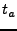, can be computed as: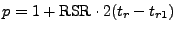(D40)

Where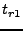is also one-way.

The one-way range time for a given pixel (number) p can be computed as: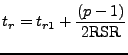(D41)

Where:
RSR is in Hz.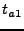is the range time to pixel 1 (first pixel) in seconds.

The range is of course equal to: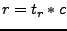(D42)

Where:
c is the speed of light (constants.h: 299792458. m/s).

Leijen 2009-04-14